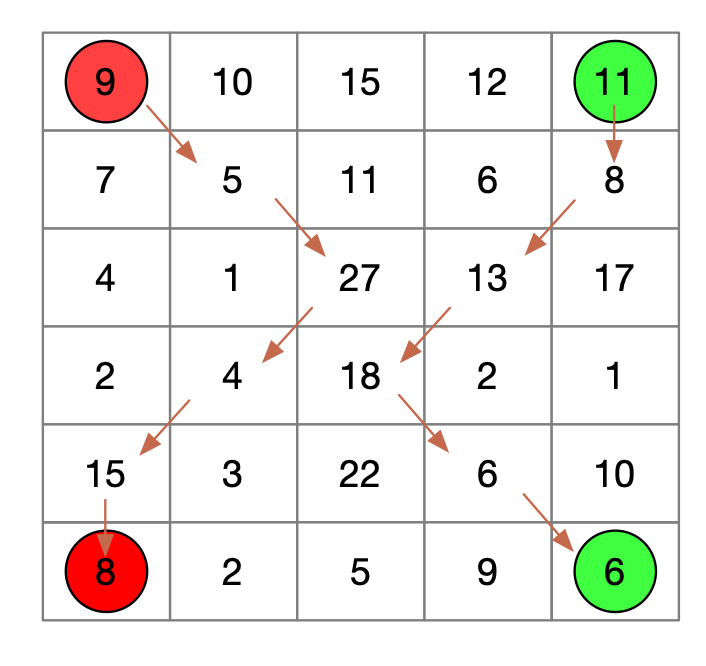# 收集硬币¶

## 1、题目描述¶

• $0<= m <= 110$
• $0<= n <= 110$
• $0<= grid[i][j] <= 12100$

6 5
9 10 15 12 11 7 5 11 6 8 4 1 27 13 17 2 4 18 2 1 15 3 22 6 10 8 2 5 9 6


1305 4
3 6 8 2 5 2 4 3 1 1 20 10 1 1 20 10 1 1 20 10


73


## 2、解题思路¶

dp[(x,y)] 表示机器人1从x点走到左下角 加上机器人2走到右下角所得到的最多的硬币数


采用倒退的方式，我们找出能够得到dp[(x,y)]的所有组合，然后利用dp[(x,y)]的值进行更新当前组合的值


def get_upper(pos):
m, n = pos // col, pos % col
temp = []
for i in [-1, 0, 1]:
if available(m - 1, n + i):
temp.append((m - 1) * col + n + i)
return temp


如果[m,n]=>[x,y]
dp[(m,n)] = max(dp[(m,n)],dp[(x,y)]+ grid[m] + grid[y] )


from collections import defaultdict
from collections import deque
from itertools import product

row, col = map(int, input().strip().split())

# 坐标验证
def available(m, n):
return 0 <= m < row and 0 <= n < col

def get_upper(pos):
m, n = pos // col, pos % col
temp = []
for i in [-1, 0, 1]:
if available(m - 1, n + i):
temp.append((m - 1) * col + n + i)
return temp

mapping_index = {index: val for index, val in enumerate(map(int, input().strip().split()))}
mem = defaultdict(int)
last_left = (row - 1) * col
last_right = row * col - 1
mem[(last_left, last_right)] = mapping_index[last_left] + mapping_index[last_right]

ans = -1

if col < 2:
ans = -1
elif col == 2:
ans = sum(map(int, input().strip().split()))
else:
d = deque()
d.append([last_left, last_right])
while d:
l, r = d.popleft()
left = get_upper(l)
right = get_upper(r)
for x, y in product(left, right):
if x != y:
mem[(x, y)] = max(mem[(x, y)], mem[(l, r)] + mapping_index[x] + mapping_index[y])
d.append([x, y])
ans = mem[(0, col - 1)]
print(ans)

#
# 6 5
# 9 10 15 12 11 7 5 11 6 8 4 1 27 13 17 2 4 18 2 1 15 3 22 6 10 8 2 5 9 6
# => 130
#

#
# 5 4
# 3 6 8 2 5 2 4 3 1 1 20 10 1 1 20 10 1 1 20 10
# => 73
#